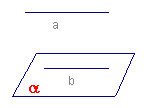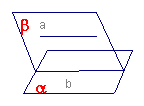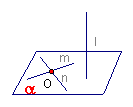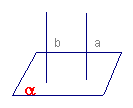## 空间直线和平面：

#### 位置关系：

（1）直线在平面内——有无数个公共点
（2）直线和平面相交——有且只有一个公共点
（3）直线和平面平行——没有公共点

#### 直线和平面平行：

• 判定定理：$a \subsetneq \alpha, b \subset \alpha, a // b \Rightarrow a // \alpha$

• 性质定理：$a// \alpha, a\subset \beta, \alpha \cap \beta = b \Rightarrow a // b$

#### 直线与平面垂直：

• 判定定理：$m \subset \alpha, n \subset \alpha, m \cap n = O, l \bot m, l \bot n \Rightarrow = l \bot \alpha$

• 性质定理：$a \bot \alpha, b \bot \alpha \Rightarrow a // b$